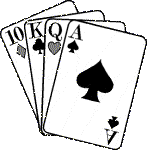## CryptoquizA Cryptoquiz is a list of words that relate to a subject--this subject being Marty Stuart. One set of letters has been substituted for the correct letters. When you've identified a word, the known letters will help you decode the other words in the group. If "M" stands for "R" in one word, the "M" will be "R" in all the words. To view the answers, click here. To help you get started, the letter "k" stands for "e". Have fun! (Another hint appears at the end of this page.)
 _ _ _ _ _ _ _ _ _ _ _ _ _ _ _ _ _ h u b e k i g y e k f c s w k g i _ _ _ _ _ _ _ d c h h b k a _ _ _ _ _ _ _ _ _ _ _ _ _ _ v o n k t h c i i d n i b s _ _ _ _ _ _ _ _ _ _ _ _ _ _ _ b e i g h n d k e g c o b i g _ _ _ _ _ _ _ _ _ _ _ _ _ _ _ _ _ _ H y s w c e a H y o o S y z v y q i _ _ _ _ _ _ _ _ _ _ _ G h c m b i G h b g g _ _ _ _ _ _ _ _ _ _ _ _ T h c e a Y o k Y l h q _ _ _ _ _ _ _ _ _ _ i y e t z h b g k h _ _ _ _ _ _ _ _ _ t h k c g u c b h _ _ _ _ _ _ _ _ _ _ _ k e g k h g c b e k h _ _ _ _ _ _ _ _ _ _ E y U c g i G y n h _ _ _ _ _ _ _ _ _ _ _ _ _ _ _ _ _ A y n v o k G h y n v o k G y n h

 Are you still stuck? Okay, another hint: The letter "g" stands for "t".